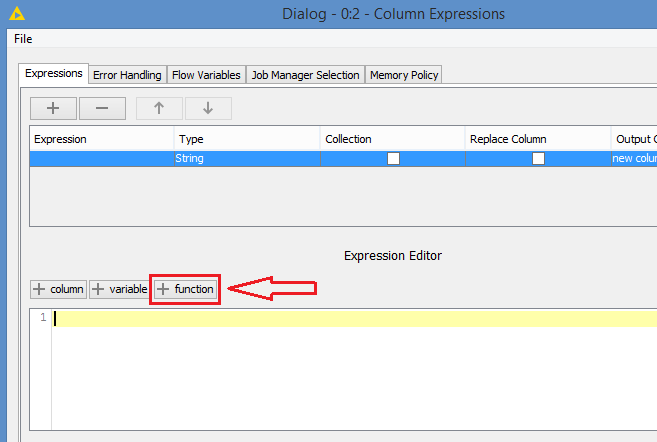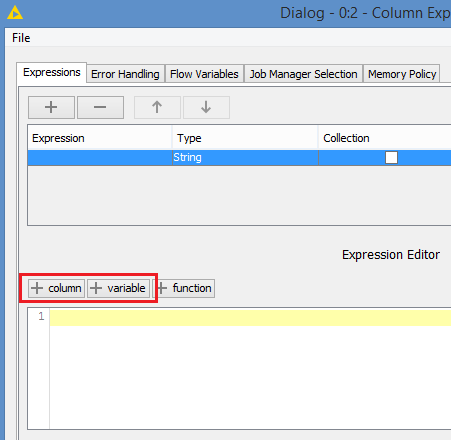# If Then Statement Conversion from Alteryx to Knime

Hello,

I need help converting an If Then Statement from Alteryx to Knime. I’ve tried the String Manipulation, Rule Engine, and Column Expression nodes but can’t seem to get it.

Which node should I use and what is the Knime syntax for the below expression?

if contains([Loc Num],"-") then “SN.”+ [Loc Num]
elseif IsNull([Loc Num]) then “”
elseif IsEmpty([Loc Num]) then “”
elseif Length([Loc Num])<=7 then “SN.”+ padleft([Loc Num],7,“0”)
elseif Length([Loc Num])>7 then “SN.”+ [Loc Num]
else “Check Formula” endif

Any help would be greatly appreciated.
Marie

1 Like

Hi @mariesylvia and welcome to the forum.

To me this definitely sounds like a job for the Column Expressions node. You can read a nice post explaining the syntax of conditionals in that node here:

1 Like

Hi @mariesylvia and welcome to the Knime Community.

Definitely go with the Column Expression node. The String Manipulation node allows you to do manipulation, but not with conditions, while the Rule Engine allows you to use conditions, but not much manipulation.

The Column Expression will allow you to do both conditions and manipulation.

The syntax to refer to a column in the Column Expression is with the column() function. In your case, if the column name is “Loc Num”, you have to reference to it with:
`column("Loc Num")`

Thank you both for the clarification on using the Column Expressions node.

I have been trying to construct my formula using the syntax mentioned but I’m still getting errors. It would be helpful to see how the below formula converts to Knime as I am pretty new at this.

if contains([Loc Num],"-") then “SN.”+ [Loc Num]
elseif IsNull([Loc Num]) then “”
elseif IsEmpty([Loc Num]) then “”
elseif Length([Loc Num])<=7 then “SN.”+ padleft([Loc Num],7,“0”)
elseif Length([Loc Num])>7 then “SN.”+ [Loc Num]
else “Check Formula” endif

Hi @mariesylvia , as I mentioned:

So, one of the things to correct is that instead of using `[Loc Num]`, you have to use `column("Loc Num")` when referring to the column “Loc Num” in Column Expression.

For syntax for if…else, you can refer to the resource that @ScottF has provided.

Hi @mariesylvia , you can check the workflow I provided in this other thread where I am using a Column Expression with if else if, and with join() which pretty much covers most of what you need.

For padleft, the synxtax is the same, except that the function is padLeft(). You can click on the function dropdown in the Column Expression to see all the available functions. You can also use the function by clicking on it in the dropdown. Similarly, you can use a column or variable by clicking on it from the column or variable dropdown.

Hello, I’ve been playing with this for a couple days now and it should be so simple but no matter what I do, I cannot get it to work. Thank you for all the help though. It is very much appreciated!

Hi @mariesylvia , We can guide you. Can you show us what you have done so far?

1 Like

@mariesylvia maybe you could provide us with some dummy data that would contain the tasks you have and also the outcome so we could develop a solution and you can check it. I am sure the forum will be able to help.

Would you do this for one specific column or several ones. Since KNIME is based on Java you always have the full power of Java at your fingertips. It might be a little bit of try and error first but you will then have the full range of options - and the KNIME forum is always there to help.

Hi
Attached is a sample of the data.

• Column A is the raw data
• Column B is the transformed data (desired outcome)

Also, below is the formula from Alteryx that needs to be converted to Knime

if contains([Loc Num],"-") then “SN.”+ [Loc Num]
elseif IsNull([Loc Num]) then “”
elseif IsEmpty([Loc Num]) then “”
elseif Length([Loc Num])<=7 then “SN.”+ padleft([Loc Num],7,“0”)
elseif Length([Loc Num])>7 then “SN.”+ [Loc Num]
else “Check Formula” endif

Data Example.xlsx (10.0 KB)

Hello,

Thank you for all the help and information.
I’m not very familiar with java but below is my latest attempt at the formula using the column expressions node. I know there are several errors but was hoping someone could help troubleshoot.

if LIKE column(“Loc Num”) “-”) {
join(“SN.”, column(“Loc Num”))
} else {
if MISSING column(“Loc Num”) {
“”
} else {
if Empty column(“Loc Num”) {
“”
} else {
if length(column(“Loc Num”) <= 7) {
} else {
if length(column(“Loc Num”) > 7) {
join(“SN.”, column(“Loc Num”)) {
} else {
“Check Formula”
}

This code works in the Column Expressions node.
if(contains(column(“Loc Num”),"-")) “SN.”+column(“Loc Num”)
else if (isNaN(column(“Loc Num”))) “”
else if (isMissing(column(“Loc Num”))) “”
else if (length(column(“Loc Num”)<=7)) “SN.” + padLeft(column(“Loc Num”), 7,“0” )
else if (length(column(“Loc Num”)>7)) “SN.” + (column(“Loc Num”), 7,“0” )
else “Check formula”

Hi @mariesylvia , both @ScottF and I could have written the correct Knime code for you in our initial posts, but the idea was to guide you enough for you to figure it out. That way you would know how to translate other future codeI’m glad to see that you are trying to get the correct translated code, it’s almost thereI’m glad you figured out how to reference to a column, but using the column() function, and you also figured out the join() function.

So, a few corrections to apply to your code:
i) if conditions should be put in brackets. For example:
`if length(column(“Loc Num”) <= 7)`

should be written as:
`if (length(column(“Loc Num”) <= 7))`

ii) An if statement can only have 1 else. It can, however, have multiple else if. The else is basically the default operation when none of the if and else if are not satisfied. It should be last. This is the same for all programming languages, and same as what you have in your Alteryx code.

The basic template would be:

``````if (condition 1) {
operation for condition 1
} else if (condition 2) {
operation for condition 2
} else if (condition n) {
operation for condition n
} else {
default operation
}
``````

Note: Parentheses are not necessary when you have just 1 operation, but it is good practice to use them. I’ve seen coders do this:

``````<some code>
if (condition)
//  operation
<some other code>
``````

They would comment out the operation part as a way to skip the if statement. However, they would not get the expected results, and would go nuts trying to figure out why. The issue here is by commenting out the operation, the `<some other code>` part now becomes the new operation, and now instead of always executing the `<some other code>`, it’s executed only if the if condition is true. With parentheses, you avoid this issue

``````<some code>
if (condition) {
//  operation
}
<some other code>
``````

`<some other code>` remains outside of the if and will execute no matter the if condition.

Note 2: Single line if statements are also accepted, like you wrote in your code.

iii) Lastly, there are a few operations that you are performing that are invalid in the Column Expression, at least it’s not the proper command. For example, you cannot use LIKE or MISSING or Empty (these are valid in other nodes). The correct replacements are actually functions that are close to what you use in your code for Alteryx.

To see what the proper function names to use, you can view the list of the functions from the function dropdown as I mentioned before. This is what I am referring to:When you click on this, you get a dropdown with all the functions that are supported. You even get a brief description of the function and how to use it:

You also don’t need to type the function in your code. You can just double click on it from the dropdown and it will appear in your code.

Similarly for accessing columns and variables, you can click on these:So, for your function `contains()`, you can use `contains()` in the Column Expression. It’s the same function.
In terms of missing and empty, if I’m not mistaken, they mean the same in Knime, and can be checked using the `isMissing()` function
And your `Length()` function is the `length()` function in Knime.

FYI, these 2 elseif from your original code:

``````elseif IsNull([Loc Num]) then “”
elseif IsEmpty([Loc Num]) then “”
``````

can be merged as 1 elseif like this in Alteryx:
`elseif IsNull([Loc Num]) OR IsEmpty([Loc Num]) then “”`

So, can you correct your if statements based on these instructions?3 Likes

Hello,

Thanks to all your help and guidance, I was able to figure out the formula!

if (contains(column(“Loc Num”),"-")) {
join(“SN.”, column(“Loc Num”))
} else if (isMissing(column(“Loc Num”))) {
“”
} else if (length(column(“Loc Num”) <= 7)) {
} else if (length(column(“Loc Num”) > 7)) {
join(“SN.”, column(“Loc Num”))
} else {
“Check Formula”
}

I really appreciate everyone’s support and patience.
It is amazing to have such a friendly, helpful, and responsive community!
Thank you!

2 Likes

Looks good to me @mariesylviaEDIT: Just a note (and that’s applicable in Alteryx or other languages too):

``````} else if (length(column(“Loc Num”) <= 7)) {
} else if (length(column(“Loc Num”) > 7)) {
join(“SN.”, column(“Loc Num”))
``````

You don’t have to do the check on the length in this case, you can just apply this no matter the length:
`join(“SN.”, padLeft(column(“Loc Num”),7 ,“0” ))`

The padding of “0” will happen only if the length is < 7. If it’s >= 7 it will not add the 0. This is all done automatically.

For example:
padLeft(“12345”, 7, “0”) will give you: 0012345 (so case where length is < 7)
padLeft(“1234567”, 7, “0”) will give you the original value: 1234567 (so case where length = 7)
padLeft(“12345678”, 7, “0”) will give you the original value: 12345678 (so case where length > 7)

Thank you for the helpful tip and again thank you for all your guidance!
I appreciate you having me solve it myself and providing guidance along the way rather than giving the answer up front. You were 100% correct that it really helps to learn how to do the formulas.

This topic was automatically closed 90 days after the last reply. New replies are no longer allowed.